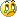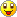Posted in Vedic Mathematics

The beauty of multiplication of 1’s with 1’s

1 x 1 = 1
11 x 11 = 1 2 1
111 x 111 = 12321
1111 x 1111 = 1234321
11111 x 11111 = 123454321
111111 x 111111 = 12345654321
1111111 x 1111111 = 1234567654321
11111111 x 11111111 = 123456787654321
111111111 x 111111111 = 12345678987654321
Observe it carefully! This is a simple logic of vedic mathematics.The multipilcation of number of one’s with the same number of one’s can be easily written.If there are 2 one’s in that number say (eleven ,has 2 one’s in it and if eleven has to be multiplied with eleven) then write first 2  natural numbers and then leave the last number and again write those numbers in reverse order.
That is,
11 x 11 = ?
step 1:
write first 2  natural numbers
1 2
step 2:then  leave the last number…that is 2 is left
step 3: again write those numbers in reverse order. that is 1
so
step 4: assemble all these 1 2 1
therefore, 11 x 11 = 1 2 1
Another example:

111 x 111
step 1: 1 2 3
step 2: leave 3
step 3:  21
step 4: assemble all 12321
therefore 111 x 111 = 12321

Will feel happy if atleast one of u understood what I wrote.I learnt this from my Sir (who was 80 years old then)